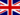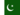¡Este curso ha sido revisado!

Para una experiencia de aprendizaje más agradable, le recomendamos que estudie la versión reeditada para móviles de este curso.

Llévame al curso revisado

- or -

Continue studying this course

Calcular el diámetro del campo visual

• Apuntes
• Revisión por temaCharles M. 0 0 The diameter of a field of view can be calculated. The information you need to do this calculation is: The size of an object in a magnified picture must be determined mathematically. To do this calculation you need to know: * the magnification of the field of view that you are interested in - magnification (new); * the size of the field of view at another magnification - field of view (old), and ; * the other magnification (magnification - old). These values are used in the formulaMUZAFAR I. 0 0 what is the formula to calculate diameter?Camile V. 0 0 Are there digital microscopes that can automatically display the magnification size? This would simplify the process since you must calculate the magnification and then go on to calculating the diameter, and finally, the field of view.Deona N. 0 0 the calls that are in your body and your blood is keepingERIC R. 0 0 Can these measurement's be reordered, calculated, stored automatically?Luciana W. 0 0 Does this mean that you have to know what size magnification you want before you can go further?